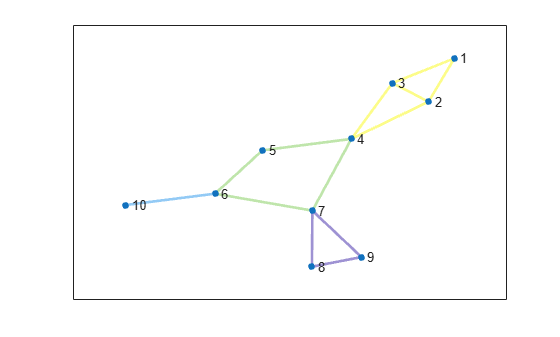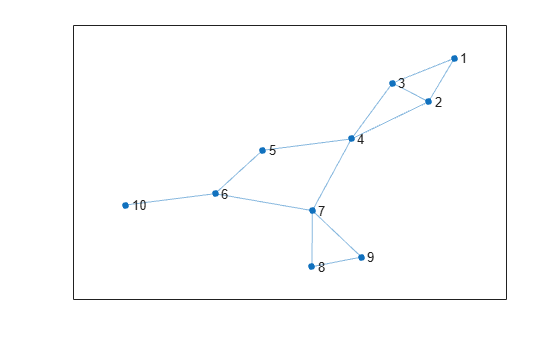# biconncomp

Biconnected graph components

## Syntax

``bins = biconncomp(G)``
``bins = biconncomp(G,'OutputForm',form)``
``````[bins,iC] = biconncomp(___)``````

## Description

example

````bins = biconncomp(G)` returns the biconnected components of graph `G` as bins. The bin numbers indicate which biconnected component each edge in the graph belongs to. Each edge in `G` belongs to a single biconnected component, whereas the nodes in `G` can belong to more than one biconnected component. Two nodes belong to the same biconnected component if removing any one node from the graph does not disconnect them.```

example

````bins = biconncomp(G,'OutputForm',form)`, where `form` is `'cell'`, returns the output as a cell array such that `bins{j}` contains the node IDs of all nodes in component `j`. The default for `form` is `'vector'`.```

example

``````[bins,iC] = biconncomp(___)``` additionally returns the node indices `iC` indicating which nodes are cut vertices (also called articulation points).```

## Examples

collapse all

Create and plot a graph. Color the edges based on which biconnected component each edge belongs to.

```s = [1 1 2 2 3 4 4 5 6 6 7 7 8]; t = [2 3 3 4 4 5 7 6 7 10 8 9 9]; G = graph(s,t); p = plot(G,'LineWidth',2);````p.EdgeCData = biconncomp(G);`This example shows how to extract the biconnected components from a graph as subgraphs, and then label the nodes in each subgraph using the node indices in the original graph.

Create and plot a graph.

```s = [1 1 2 2 3 4 4 5 6 6 7 7 8]; t = [2 3 3 4 4 5 7 6 7 10 8 9 9]; G = graph(s,t); plot(G)```Group the graph nodes into bins based on which biconnected component(s) each node belongs to. Then, loop through each of the bins and extract a subgraph for each biconnected component. Label the nodes in each subgraph using their original node indices.

```bincell = biconncomp(G, 'OutputForm', 'cell'); n = length(bincell); for ii = 1:n subplot(2,2,ii) plot(subgraph(G, bincell{ii}), 'NodeLabel', bincell{ii}); end```Identify the cut vertices in a graph and then highlight those vertices in the graph plot.

Create and plot a graph. Calculate which biconnected component each graph edge belongs to, and specify a second output to return a vector identifying the cut vertices.

```s = [1 1 2 2 3 4 4 5 6 6 7 7 8]; t = [2 3 3 4 4 5 7 6 7 10 8 9 9]; G = graph(s,t); p = plot(G);````[edgebins,iC] = biconncomp(G)`
```edgebins = 1×13 4 4 4 4 4 3 3 3 3 2 1 1 1 ```
```iC = 1×3 4 6 7 ```

Nodes 4, 6, and 7 are the cut vertices of graph `G`. Use `highlight` to enlarge the cut vertices referenced in `iC`.

`highlight(p, iC)`## Input Arguments

collapse all

Input graph, specified as a `graph` object. Use `graph` to create an undirected graph object.

Example: `G = graph(1,2)`

Type of output, specified as one of these values:

OptionOutput
`'vector'` (default)`bins` is a numeric vector indicating which biconnected component each edge belongs to.
`'cell'``bins` is a cell array, and `bins{j}` contains the node IDs for all nodes that belong to component `j`.

## Output Arguments

collapse all

Biconnected components, returned as a vector or cell array. The bin numbers assign each edge or node in the graph to a biconnected component:

• If `OutputForm` is `'vector'` (default), then `bins` is a numeric vector indicating which connected component (bin) each edge belongs to. Edges that are self-loops are assigned to bin `0`, since they do not belong to any biconnected component.

• If `OutputForm` is `'cell'`, then `bins` is a cell array, with `bins{j}` containing the node IDs for all nodes that belong to component `j`.

Indices of cut vertices, returned as a vector of numeric node IDs.

collapse all

### Biconnected Components

A biconnected component of a graph is a maximally biconnected subgraph. A graph is biconnected if it does not contain any cut vertices.

Decomposing a graph into its biconnected components helps to measure how well-connected the graph is. You can decompose any connected graph into a tree of biconnected components, called the block-cut tree. The blocks in the tree are attached at shared vertices, which are the cut vertices.

The illustration depicts:

• (a) An undirected graph with 11 nodes.

• (b) Five biconnected components of the graph, with the cut vertices of the original graph colored for each component to which they belong.

• (c) Block-cut tree of the graph, which contains a node for each biconnected component (as large circles) and a node for each cut vertex (as smaller multicolored circles). In the block-cut tree, an edge connects each cut vertex to each component to which it belongs.### Cut Vertices

Also known as articulation points, cut vertices are graph nodes whose removal increases the number of connected components. In the previous illustration, the cut vertices are those nodes with more than one color: nodes 4, 6, and 7.

## Version History

Introduced in R2016b